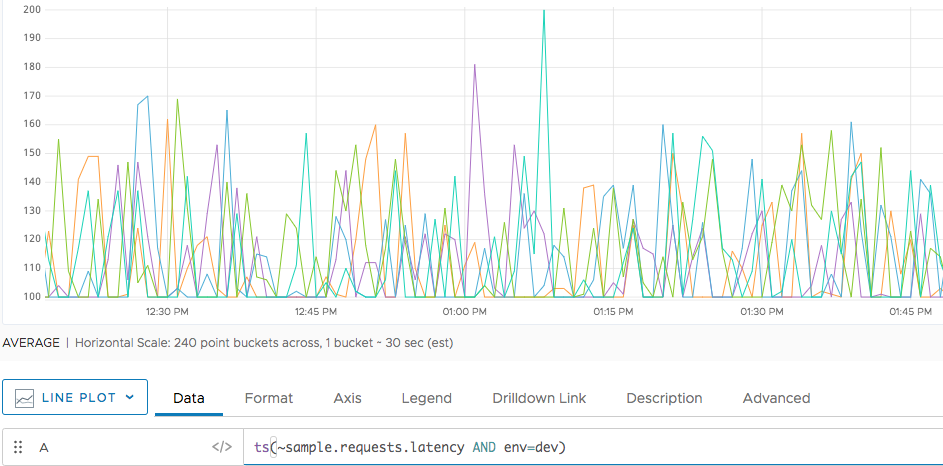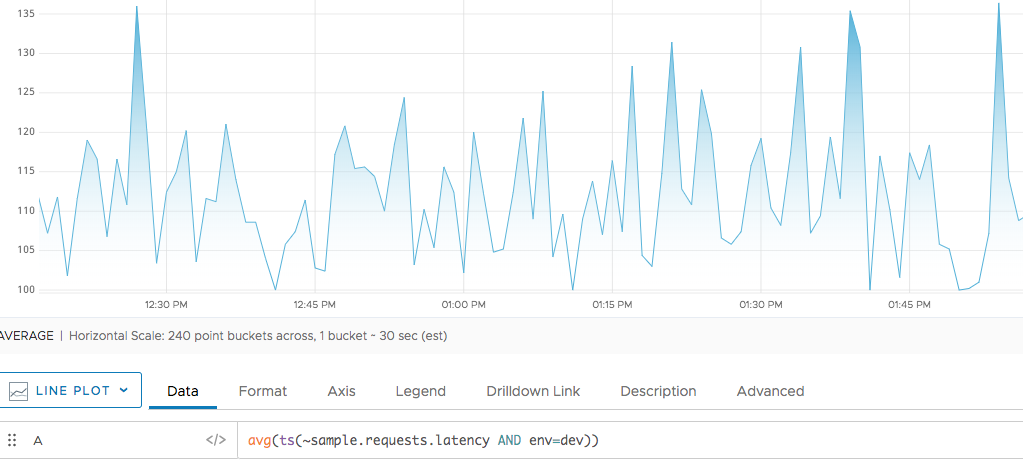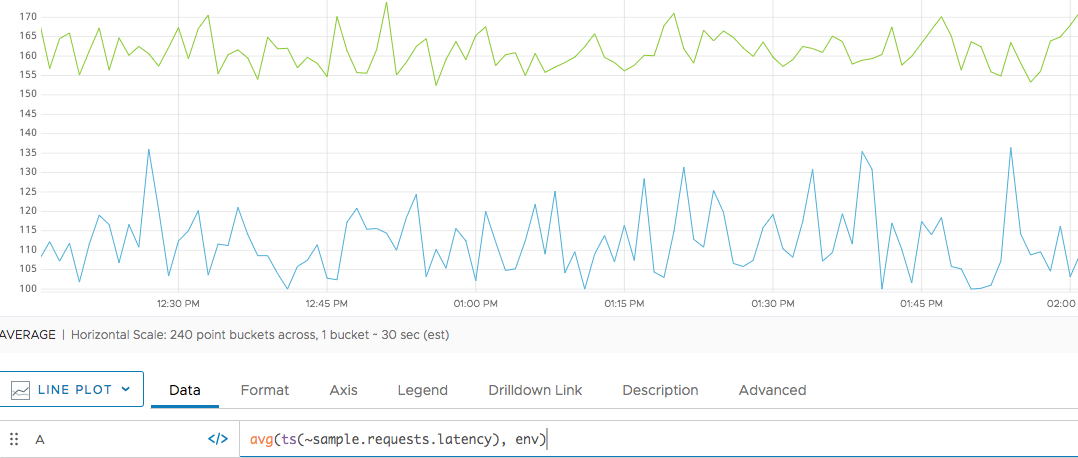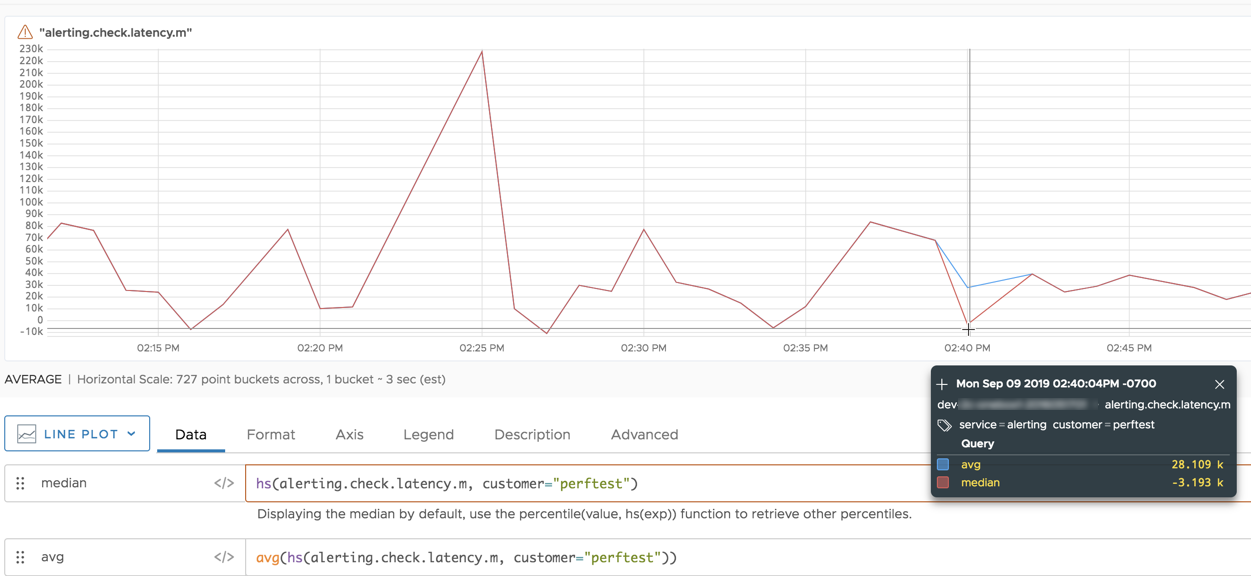Reference to the avg() function

## Summary

``````avg(<tsExpression>[, metrics|sources|sourceTags|pointTags|<pointTagKey>])

avg(<hsExpression>)
``````

You can use `avg()` with time series and with histograms.

 Time series aggregation function Returns the average (the mean) of the set of time series described by the expression. The results might be computed from real reported values and interpolated values. Use rawavg() if you don't need interpolation. Histogram conversion function Returns time series that consist of the average value from each histogram distribution described by the `hsExpression`.

## Parameters

### Time-Series Aggregation Function

ParameterDescription
tsExpression Expression describing the set of time series to be averaged.
metrics|sources|sourceTags|pointTags|<pointTagKey> Optional 'group by' parameter for organizing the time series into subgroups and then returning the average for each subgroup. Use one or more parameters to group by metric names, source names, source tag names, point tag names, values for a particular point tag key, or any combination of these items. Specify point tag keys by name.

### Histogram Conversion Function

ParameterDescription
hsExpression Expression describing the histogram series to obtain average values from.

## Description

You can use `avg()`:

• With time series as an aggregation function.
• With histogram series as a conversion function.

### Time-Series Aggregation Function

The `avg()` aggregation function averages the data values at each moment in time, across the time series that are represented by the expression.

By default, `avg()` produces a single series of averages by aggregating values across all time series. You can optionally group the time series based on one or more characteristics, and obtain a separate series of averages for each group.

If any time series has data gaps, `avg()` fills them in by interpolation whenever possible.

The `avg()`, `mavg()` and `mmedian()` functions can help you understand the tendency of the data.

• Use `avg()` or `mavg()` to get the mean (average), that is, the number in the middle of a set of values.
• Use `mmedian()` to be less sensitive to outliers. Even a single outlier can affect the result of `avg()` and `mavg()`. Use `mpercentile()` with a percentile of 50 to get the moving median.

#### Grouping

Like all aggregation functions, `avg()` returns a single series of results by default. You can include a ‘group by’ parameter to obtain separate averages for groups of time series that share common metric names, source names, source tags, point tags, or values for a particular point tag key. The function returns a separate series of results corresponding to each group.

You can specify multiple ‘group by’ parameters to group the time series based on multiple characteristics. For example, `avg(ts("cpu.cpu*"), metrics, Customer)` first groups by metric names, and then groups by the values of the `Customer` point tag.

#### Interpolation

If any time series has gaps in its data, the query engine attempts to fill these gaps with interpolated values before applying the function. A value can be interpolated into a time series only if at least one other time series reports a real data value at the same moment in time.

Within a given time series, an interpolated value is calculated from two real reported values on either side of it. Sometimes interpolation is not possible–for example, when a new value has not been reported yet in a live-view chart. In this case, the query engine finds the last known reported value in the series, and assigns it to any subsequent moment in time for which a real reported data value is present in some other time series. We use the last known reported value only if interpolation can’t occur and if the last known reported value has been reported within the last 15% of the query time in the chart window.

You can use `rawavg()` to suppress interpolation. See Standard Versus Raw Aggregation Functions.

### Histogram Conversion Function

The `avg()` histogram conversion function computes the average of the data values in each distribution of a histogram series that is represented by the expression. The averages for a given histogram series are returned as a separate time series that contains a data point corresponding to each input distribution.

`avg()` is a histogram conversion function because it takes histogram distributions as input, and returns time series. You can therefore use a histogram conversion function as a `tsExpression` parameter in a time series query function.

## Examples

### Time-Series Aggregation Function

The following example shows the data for `sample.requests.loadavg`. To limit the number of lines, we’re filtering to show only time series with `env=dev`.When we apply `avg()` we get a single line.We can group by the `env()` point tag to see the differences between the dev and production servers.### Histogram Conversion Function

In the following example, the blue line shows the result of applying `avg()` to an `hsExpression`. In this particular example, the average is nearly always the same as the median (the red line), with some divergent values around 2:40p.Note: `avg()` returns a separate time series for each input histogram series. In this example, the `hsExpression` represents a single histogram series, so the result is a single time series. (In contrast, when `avg()` is applied to a `tsExpression`, a single returned time series might be the result of combining multiple input time series.)

## Caveats for Time Series

Using `rawavg()` instead of `avg()` can significantly improve query performance because `rawavg()` does not perform interpolation.